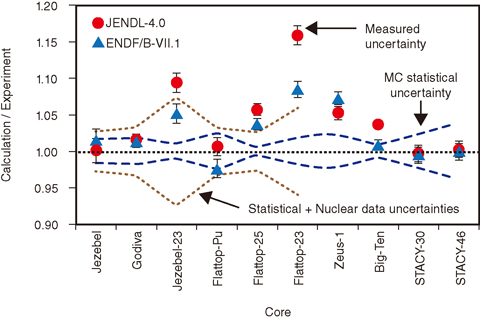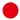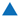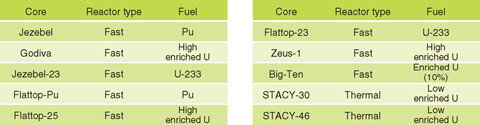# 4-1 Highly Accurate Estimation of Nuclear Reactor Parameters

## －Development of a Novel Calculation Method for Calculating Reactor Kinetics Parameters with Monte Carlo Method－Fig.4-3　Comparison of calculated and measured values

Measured α values at a delayed critical condition and calculated α values with the MVP code are shown for various critical assemblies (Table 4-1).andshow the results obtained with the nuclear data library JENDL-4.0 developed at JAEA and the U.S. library ENDF/B-VII.1, respectively; the error bars denote experimental uncertainties. The uncertainties induced by nuclear data are estimated for the six assemblies.

Table 4-1　Critical assemblies for which reactor kinetics parameters have been calculatedThis Picture(117KB)

The Monte Carlo (MC) method used for reactor analysis simulates the behavior of neutrons in a reactor to calculate nuclear reactor parameters. This method can model the calculation geometry very accurately, compared with the deterministic method conventionally used for reactor design calculations. In addition, the cross-section data, which represent the probability of the interaction between a neutron and a nucleus, can be addressed continuously as a function of energy. In other words, the MC method enables us to perform highly accurate calculations with almost no approximations.

The MC method has great advantages, but one cannot calculate all the parameters necessary for nuclear reactor analysis. The parameters that one cannot calculate to date using the MC method are the reactor kinetics parameters, the effective delayed neutron fraction, and the neutron-generation time. These are nuclear safety parameters directly related to reactor power variation. Thus, these parameters must be calculated as accurately as possible because small changes in them significantly affect the reactor power.

We have developed a novel method to for calculating the reactor kinetics parameters with the MC method. The difficulty lies in defining the parameters, which is done using the ratio of adjoint-weighted values. The novel method does not calculate adjoint-weighted values but does exactly calculate the parameters with the differential coefficient of the effective multiplication factor. To verify the novel method, we have implemented it into our MC code MVP and have compared the α value under a delayed critical condition for various critical assemblies (Table 4-1). Fig.4-3 shows a comparison between the calculated and experimental α values. The α value at the delayed critical condition is defined by the effective delayed neutron fraction divided by the neutron generation time and multiplied by −1; the α value is a nuclear reactor parameter that can be measured with critical experiments. The calculated values agree fairly well with the measured ones within the MC statistical and nuclear-data-induced uncertainties; there are some calculated values that significantly differ from the measured ones.

The outcome of this research enables us to calculate the reactor kinetics parameters. It is expected that the novel method can provide reference values for the parameters for nuclear systems including fuel debris and innovative reactors of new types.

| | | | |